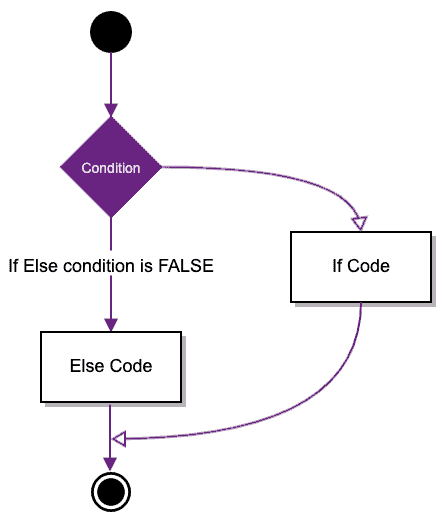# R If else Statement (With Examples)

The “if else” statement in R evaluates a condition and executes different statements based on whether the condition is TRUE or FALSE.

The syntax of if-else is:

``````if (condition) {
expression A
} else {
expression B
}``````

Here, the “condition” is an expression that evaluates to TRUE or FALSE. If the condition is TRUE, the code within the first set of braces is executed, and if the condition is FALSE, the code within the second set of braces is executed.

For example, if you want to print “R Language” when x is greater than 21 and “Python” otherwise, you can write:

``````if (x > 21) {
print("R Language")
} else {
print("Python")
}``````

## Flow chart of if-else in R## if statement in R

See the following syntax of the if statement in R.

``````if (condition) {
expression
}``````

If the condition is TRUE, the statement gets executed. But if it’s FALSE, then nothing will happen.

Only the first item is considered if the condition can be a logical or numeric vector.

In the case of a numeric vector, zero is taken as FALSE; the rest is TRUE.

``````qunatity <- 12

if (qunatity > 0) {
print("You need to purchase dozen")
}``````

Output

`` "You need to purchase dozen"``

## if..else if..else if..else statement

The “if statement” can be followed by the optional else if…else statement, which is beneficial to test various conditions using the single if…else if statement.

Remember a few points when using if-else, if, and else statements.

1. If statement can have zero or one else, it must come after any else if’s.
2. If statement can have zero to many else if’s, they must come before the else.
3. Once an else-if succeeds, None of the remaining if’s or else’s will be tested.

The if…else ladder (if…else…if) statement allows you to execute a code block among more than 2 alternatives.

The syntax of the if…else statement is:

``````if (condition) {
expressions A
} else if (condition) {
expressions B
} else if (condition) {
expressions C
} else {
expressions D
}``````

See the below example of the if-else ladder in R.

``````quantity <- 12

if (quantity < 0) {
print("It is not valid")
} else if (quantity == 6) {
print("It is half dozen")
} else if (quantity == 12) {
print("It is a dozen")
} else {
print("It is a more than dozen")
}
``````

Output

`` "It is a dozen"``

If the quantity is negative, then if block will be executed. For example, if the quantity is 6, the first else-if block is executed. If the quantity is 12, the second else-if block is executed; otherwise, the last else block is executed.

## Conclusion

The “if-else” statement in R evaluates a condition and executes different statements based on whether the condition is TRUE or FALSE.